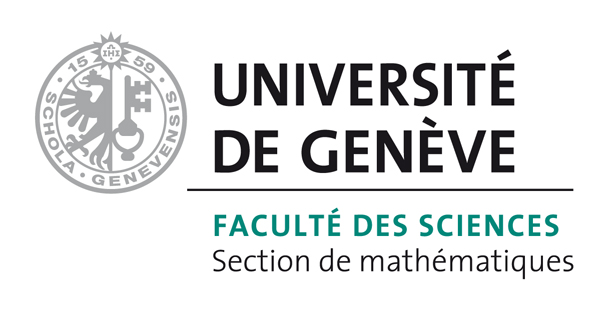# Bart Vandereycken## Criss-cross type algorithms for computing the real pseudospectral abscissa

by ,

#### Abstract:

The real ε-pseudospectrum of a real matrix A consists of the eigenvalues of all real matrices that are ε-close to A. The closeness is commonly measured in spectral or Frobenius norm. The real ε-pseudospectral abscissa, which is the largest real part of these eigenvalues for a prescribed value ε, measures the structured robust stability of A. In this paper, we introduce a criss-cross type algorithm to compute the real ε-pseudospectral abscissa for the spectral norm. Our algorithm is based on a superset characterization of the real pseudospectrum where each criss and cross search involves solving linear eigenvalue problems and singular value optimization problems. The new algorithm is proved to be globally convergent, and observed to be locally linearly convergent. Moreover, we propose a subspace projection framework in which we combine the criss-cross algorithm with subspace projection techniques to solve large-scale problems. The subspace acceleration is proved to be locally superlinearly convergent. The robustness and efficiency of the proposed algorithms are demonstrated on numerical examples.

#### Reference:

D. Lu, B. Vandereycken, "Criss-cross type algorithms for computing the real pseudospectral abscissa", In SIAM J. Matrix Anal. Appl., vol. 38, no. 3, pp. 891-923, 2017.

#### Bibtex Entry:

@article{Lu_V:2017,
Abstract = {The real ε-pseudospectrum of a real matrix A consists of the eigenvalues of all real matrices that are ε-close to A. The closeness is commonly measured in spectral or Frobenius norm. The real ε-pseudospectral abscissa, which is the largest real part of these eigenvalues for a prescribed value ε, measures the structured robust stability of A. In this paper, we introduce a criss-cross type algorithm to compute the real ε-pseudospectral abscissa for the spectral norm. Our algorithm is based on a superset characterization of the real pseudospectrum where each criss and cross search involves solving linear eigenvalue problems and singular value optimization problems. The new algorithm is proved to be globally convergent, and observed to be locally linearly convergent. Moreover, we propose a subspace projection framework in which we combine the criss-cross algorithm with subspace projection techniques to solve large-scale problems. The subspace acceleration is proved to be locally superlinearly convergent. The robustness and efficiency of the proposed algorithms are demonstrated on numerical examples.},
Author = {Lu, D. and Vandereycken, B.},
Journal = {SIAM J. Matrix Anal. Appl.},
Title = {Criss-cross type algorithms for computing the real pseudospectral abscissa},
Number = {3},
Pages = {891--923},
Volume = {38},
Doi = {10.1137/16M107952X},
Pdf = {http://www.unige.ch/math/vandereycken/papers/published_Lu_V_2017.pdf},
Year = 2017}